# Class 10 Maths: A Building Block For Success In Advanced Mathematics

###### ByRamraj Saini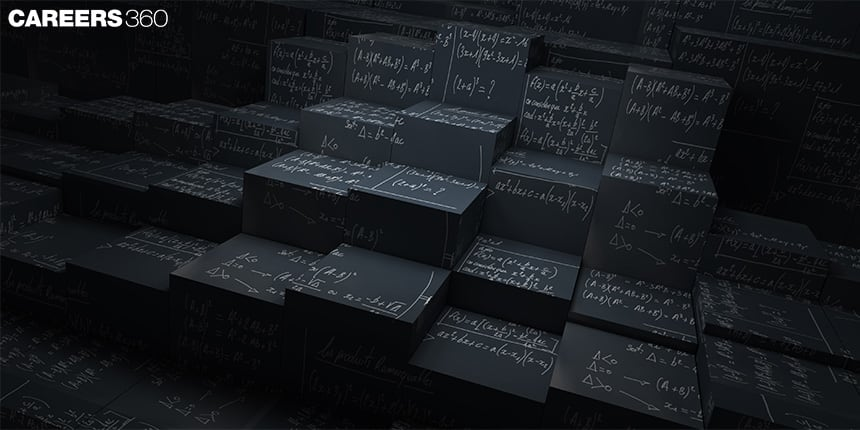###### Synopsis

Class 10 Maths is crucial in a student's mathematical journey as it lays the foundation for advanced maths courses such as calculus, differential equations, vector algebra, three-dimensional geometry, and more such concepts. Therefore, having a strong understanding of the subject is crucial. Also, many fields, such as engineering, finance, physics, and architecture, heavily rely on the mathematical concepts learned in class 10th maths.###### Synopsis

Class 10 Maths is crucial in a student's mathematical journey as it lays the foundation for advanced maths courses such as calculus, differential equations, vector algebra, three-dimensional geometry, and more such concepts. Therefore, having a strong understanding of the subject is crucial. Also, many fields, such as engineering, finance, physics, and architecture, heavily rely on the mathematical concepts learned in class 10th maths.

In the 21st century, problem-solving ability is one of the most important skills. This ability is often considered a part of design thinking, and it involves approaching problems in a logical, abstract, and lateral manner. Mathematics plays an important role in developing this ability, as it provides various methods for arriving at solutions and teaches us to choose the easiest and most efficient way to reach a correct solution. Training in mathematics not only helps to build cognitive skills but also fine-tunes them for lateral, abstract, and logical thinking. It also encourages questioning and the development of critical thinking skills.

Maths covered in Class 10 lays the foundation for advanced maths courses in the future. The concepts and problem-solving techniques learned here provide the necessary tools for understanding more complex mathematical ideas. Additionally, many fields, such as engineering, finance, and physics, heavily rely on the mathematical concepts learnt in Class 10. So let's look into the essential mathematical concepts that are the building blocks for future classes.

## Concepts That Are Building Block For Advance Maths

NCERT Class 10 Maths contains multiple concepts including algebra, geometry, trigonometry, statistics, probability, and many more. Let's discuss how these are essential for advanced maths.

### Algebra

Class 10 Algebra introduces students to the basic concepts and techniques of algebraic manipulation. It is a branch of mathematics that deals with mathematical equations and their solutions, and it is also the foundation for calculus and linear algebra. By studying Algebra, students can develop a strong understanding of mathematical equations, variables, and operations, which will serve as the foundation for more advanced mathematical concepts in future classes.

Some key concepts and techniques that students learn include solving linear equations, quadratic equations, and understanding the concept of variables. They also learn about polynomials and the various operations such as addition, subtraction, multiplication, division, and finding the roots that can be performed on them. This knowledge is useful when students move on to advanced maths classes such as calculus, where they will learn how to solve problems related to rates of change and optimisation.

### Geometry

In Class 10 students learn about basic geometric shapes like points, lines, angles, and figures, the concepts of congruence and similarity, the Pythagorean theorem, and the various formulas related to circles, triangles, and quadrilers. These concepts are the building blocks for understanding more advanced geometric concepts such as coordinate geometry, three-dimensional geometry, and vector geometry.

Coordinate geometry, for example, is a branch of mathematics that deals with the representation of geometric shapes using coordinates. It is used in fields such as engineering and physics to represent and analyse geometric shapes. Three-dimensional geometry, on the other hand, deals with the study of 3D shapes and their properties. It is used in engineering, architecture, and computer graphics to design and analyse 3D shapes.

Vector geometry is another critical concept that builds on the foundation of Class 10 Geometry. It deals with the study of mathematical vectors and their applications. It is used in engineering to represent and analyse physical quantities such as velocity and acceleration.

In addition to these concepts, class 10th geometry also introduces students to problem-solving techniques that are essential for success in advanced maths courses. For example, students learn how to use logical reasoning and critical thinking to solve geometric problems. They also learn how to use geometric formulas and equations to solve problems. These problem-solving skills are essential for success in fields such as engineering, physics, and architecture.

Furthermore, the geometry taught in Class 10 also helps students develop the ability to think out of the box and to reason logically. This is important for success in fields such as computer science, finance and business, where logical reasoning is essential for making strategic decisions.

### Trigonometry

Class 10 trigonometry provides the foundation for understanding more advanced mathematical concepts. Trigonometry is the branch of mathematics that deals with the relationship between the angles and sides of a triangle. The concepts learned in Class 10 trigonometry, such as trigonometric ratios, identities, and functions, are essential for understanding more advanced mathematical concepts such as Calculus and Physics.

In higher grades, students will encounter more advanced trigonometry concepts such as inverse trigonometric functions, trigonometric equations, and complex numbers.

Trigonometry is also used in fields such as physics, engineering, and computer science, where it is used to calculate the force and motion of objects. In physics, trigonometry is used to calculate the motion of objects in two and three dimensions. In engineering, trigonometry is used to design structures and machines. And in computer science, trigonometry is used to create graphics and animations.

### Statistics And Probability

In Class 10, students learn about basic statistical concepts such as measures of central tendency and measures of dispersion. They also learn about probability, including the concept of experimental probability, theoretical probability and conditional probability. These concepts are essential for understanding more advanced statistical techniques such as hypothesis testing and statistical inference, which are used to make decisions and predictions based on data.

Additionally, Class 10 Statistics and Probability also introduces students to the use of graphs and charts, which are commonly used to display and analyse data. By learning how to interpret and create various types of graphs, students develop important skills in data visualisation and analysis. These skills are vital for understanding and making sense of data in fields such as finance, economics, and business.

Students also learn about the concept of sampling and sampling distributions, which are used to make inferences about a population based on a sample of data. This is an important concept in fields such as market research, where it is used to make predictions about consumer behaviour and preferences.

It is important that students take their Class 10th Maths seriously and to work hard to develop a strong understanding of the concepts and problem-solving techniques taught in this class.

Also check - Oxytocin: How The Love Hormone Affects Humans

• Science
• Branches of science
• Problem solving
• Statistics
• Algebra
• Analytic geometry
• Geometry
• Trigonometry
• Mathematics
• Equation

## Careers360 helping shape your Career for a better tomorrow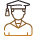#### 250M+

Students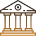#### 30,000+

Colleges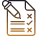#### 500+

Exams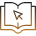E-Books